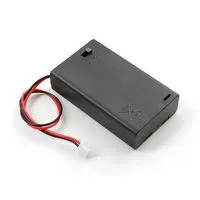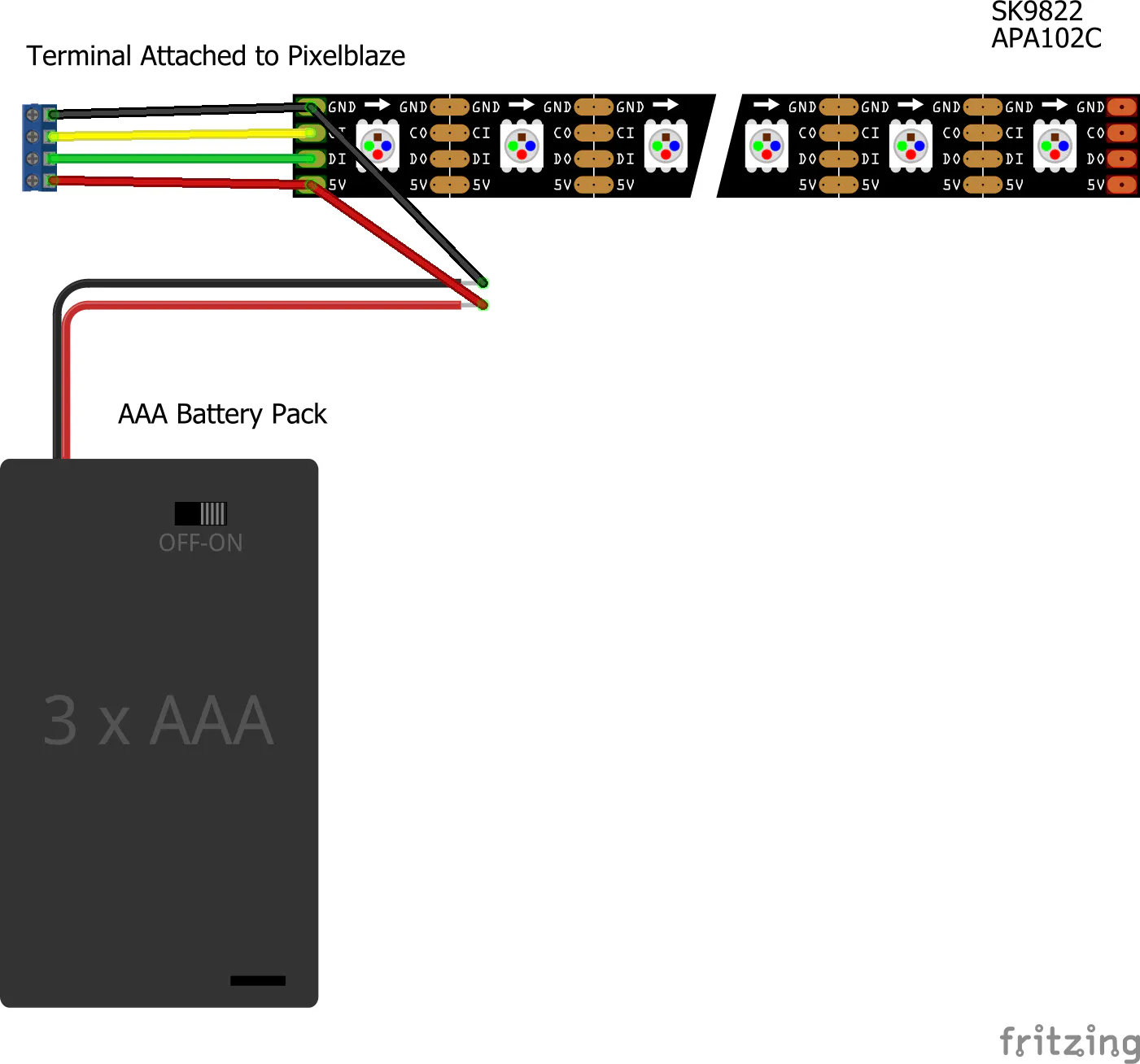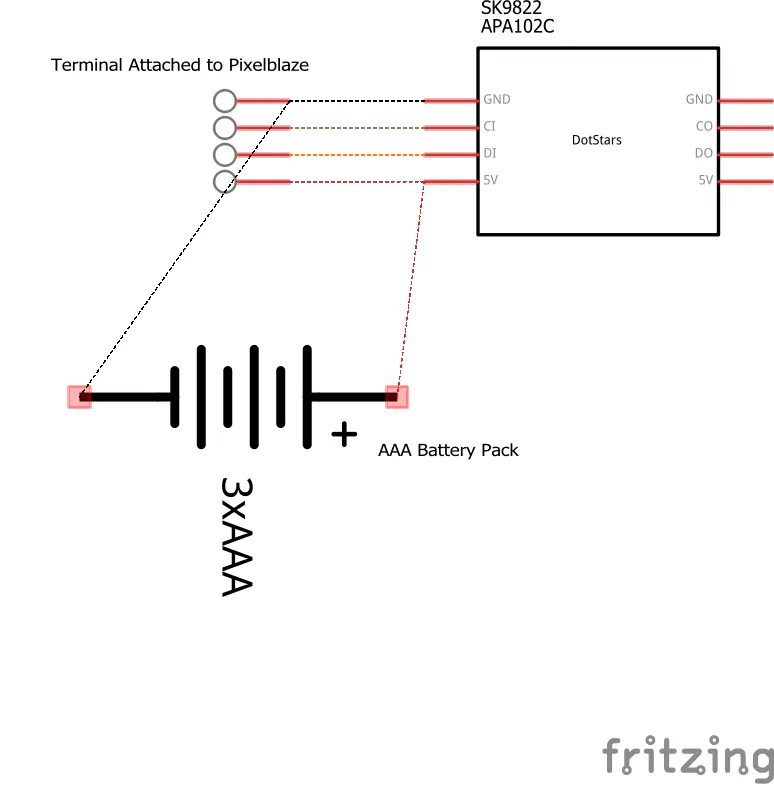# LEGO Dots Cyberpunk Belt

#LetsBuildTogether LEGO Dots wearable light-up belt.

BeginnerFull instructions provided2 hours2,073## Things used in this project

### Hardware components

 LEGO Dots
×16
 DotStar LEDs
×1
 Pixelblaze V2
×1Battery Holder, 3 x AAA
×1
 AAA Batteries
×3

### Hand tools and fabrication machinesSoldering iron (generic)
 Kester Solder

## Schematics

### SK9822 Pixelblaze Brd

You can solder the LEDs directly to the pixelblaze controller or you can solder terminals to the pixelblaze controller.### LEGO Dots Belt Schematic## Code

### Glitch Band LED Pattern

JavaScript
```export function beforeRender(delta){
t1 = time(0.1)*PI2;
t2 = time(.1);
t3 = time(.5);
t4 = time(0.1)*PI2;
t5 = time(0.05);
t6 = time(0.02);
}

export function render(index) {
h = sin(t1)
m = (.3 + triangle(t2) * .2)
h = h + (((index - pixelCount / 2 ) / pixelCount * (triangle(t3) * 10 + 4 * sin(t4)) % m))
s1 =  triangle((t5 + index / pixelCount * 5) % 1);
s1 = s1 * s1
s2 = triangle((t6 - (index - pixelCount) / pixelCount) % 1);
s2 = s2 * s2 * s2 * s2
s = 1 -triangle(s1 * s2)
v = (s1 >s2) + .5
hsv(h, s, v)
}
```

## Credits

### Amie DD

6 projects • 41 followers
Maker of Things